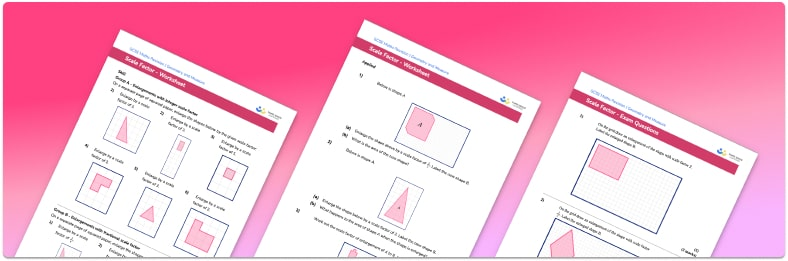# Scale Factor Worksheet• Section 1 of the scale factor worksheet contains 36 skills-based scale questions, in 3 groups to support differentiation
• Section 2 contains 4 applied scale factor problems with a mix of worded questions and deeper problem solving questions
• Section 3 contains 4 foundation and higher level GCSE exam style scale questions
• Answers and a mark scheme for all scale factor questions are provided
• Questions follow variation theory with plenty of opportunities for students to work independently at their own level
• All questions created by fully qualified expert secondary maths teachers

Suitable for GCSE maths revision for AQA, OCR and Edexcel exam boards

• This field is for validation purposes and should be left unchanged.

You can unsubscribe at any time (each email we send will contain an easy way to unsubscribe). To find out more about how we use your data, see our privacy policy.

### Scale factor at a glance

Scale in maths involves maps and other scale drawings. If something is drawn to scale, such as a map, it is an accurate representation of a bigger thing, with all distances on the map or drawing in the same proportion as in real life.

The scale factor for a map is usually given in the form 1:n , where the distances on the map are n times smaller than in real life. We can calculate missing lengths between two places by measuring the distance on the map and multiplying by n

For scale drawings, calculating scale factors can be done by dividing the real life length by the length on the drawing. In the same way, we can calculate the scale factor between two mathematically similar figures by dividing the length of a given side of shape A by the length of the corresponding side of shape B. Once we have the scale factor, it can be used to calculate the lengths of any missing sides of the shapes.

The enlargement of an object requires a scale factor where the new shape can be larger or smaller than the original.

Scale problems require students to be proficient in working with whole numbers, ratio, fractions and decimals.

Looking forward, students can then progress to additional transformations worksheets and other ratio and proportion worksheets, for example a ratio worksheet or a simplifying and equivalent ratios worksheet.

## Do you have KS4 students who need more focused attention to succeed at GCSE?There will be students in your class who require individual attention to help them succeed in their maths GCSEs. In a class of 30, it’s not always easy to provide.

Help your students feel confident with exam-style questions and the strategies they’ll need to answer them correctly with our dedicated GCSE maths revision programme.

Lessons are selected to provide support where each student needs it most, and specially-trained GCSE maths tutors adapt the pitch and pace of each lesson. This ensures a personalised revision programme that raises grades and boosts confidence.

Find out more